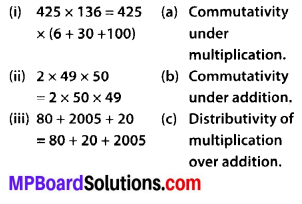## MP Board Class 6th Maths Solutions Chapter 2 Whole Numbers Ex 2.2

Question 1.
Find the sum by suitable rearrangement:
(a) 837 + 208 + 363
(b) 1962 + 453 + 1538 + 647
Solution:
(a) 837 + 208 + 363
= (837 + 363) + 208
= 1200 + 208 = 1408

(b) 1962 + 453 + 1538 + 647
= (1962 + 1538) + (453 + 647)
= 3500 + 1100 = 4600Question 2.
Find the product by suitable rearrangement:
(a) 2 × 1768 × 50
(b) 4 × 166 × 25
(c) 8 × 291 × 125
(d) 625 × 279 × 16
(e) 285 × 5 × 60
(f) 125 × 40 × 8 × 25
Solution:
(a) 2 × 1768 × 50
= (2 × 50) × 1768 – 100 × 1768
= 176800

(b) 4 × 166 × 25
= (4 × 25) × 166
= 100 × 166
= 16600

(c) 8 × 291 × 125
= (8 × 125) × 291
= 1000 × 291
= 291000

(d) 625 × 279 × 16
= (625 × 16) × 279
= 10000 × 279
= 2790000

(e) 285 × 5 × 60
= 285 × (5 × 60)
= 285 × 300
= 85500

(f) 125 × 40 × 8 × 25
= (125 × 8) × (40 × 25)
= 1000 × 1000
= 1000000

Question 3.
Find the value of the following:
(a) 297 × 17 + 297 × 3
(b) 54279 × 92 + 8 × 54279
(c) 81265 × 169 – 81265 × 69
(d) 3845 × 5 × 782 + 769 × 25 × 218
Solution:
(a) 297 × 17 + 297 × 3
= 297 × (17 + 3)
= 297 × 20
= 5940

(b) 54279 × 92 + 8 × 54279
= 54279 × (92 + 8)
= 54279 × 100
= 5427900

(c) 81265 × 169 – 81265 × 69
= 81265 × (169 – 69)
= 81265 × 100
= 8126500

(d) 3845 × 5 × 782 + 769 × 25 × 218
= 3845 × 5 × 782 + 769 × 5 × 5 × 218
= 3845 × 5 × 782 + 3845 × 5 × 218
= 3845 × 5 × (782 + 218)
= 19225 × 1000
= 19225000Question 4.
Find the product using suitable properties.
(a) 738 × 103
(b) 854 × 102
(c) 258 × 1008
(d) 1005 × 168
Solution:
By using distributive property of multiplication over addition, we get
(a) 738 × 103
= 738 × (100 + 3)
= 738 × 100 + 738 × 3
= 73800 + 2214 = 76014

(b) 854 × 102
= 854 × (100 + 2)
= 854 × 100 + 854 × 2
= 85400 + 1708
= 87108

(c) 258 × 1008
= 258 × (1000+ 8)
= 258 × 1000 + 258 × 8
= 258000 + 2064
= 260064

(d) 1005 × 168
= (1000 + 5) × 168
= 1000 × 168 + 5 × 168
= 168000 + 840
= 168840Question 5.
A taxidriver filled his car petrol tank with 40 litres of petrol on Monday. The next day, he filled the tank with 50 litres of petrol. If the petrol costs ₹ 44 per litre, how much did he spend in all on petrol?
Solution:
Petrol filled on Monday = 40 litres Petrol filled on next day = 50 litres
Total petrol filled = (40 + 50) litres = 90 litres
Now, cost of 1 litre of petrol = ₹ 44
Cost of 90 litres of petrol = ₹ (44 × 90)
= ₹ 3960
Therefore, the taxidriver spent ₹ 3960 on petrol.

Question 6.
A vendor supplies 32 litres of milk to a hotel in the morning and 68 litres of milk in the evening. If the milk costs ₹ 15 per litre, how much money is due to the vendor per day?
Solution:
Supply of milk in the morning = 32 litres
Supply of milk in the evening = 68 litres
Total supply of milk = (32 + 68) litres
= 100 litres
Now, cost of 1 litre milk = ₹ 15
Cost of 100 litres milk = ₹ (15 × 100)
= ₹ 1500
Therefore, ₹ 1500 is due to the vendor per day.Question 7.Solution:
(i) 425 × 136 = 425 × (6 + 30 + 100)
This is the principle of distributivity of multiplication over addition.

(ii) 2 × 49 × 50 = 2 × 50 × 49
This is the principle of commutativity under multiplication.

(iii) 80 + 2005 + 20 = 80 + 20 + 2005
This is the principle of commutativity under addition.